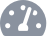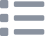# Thermistor Sensor Module with micro:bit

## Learn to use a thermistor module with micro:bit

Written By: Cherie TanDifficulty
EasySteps
11

### Introduction

A thermistor changes its resistance with temperature.

In this guide, learn to use a thermistor module with the micro:bit and program it in the MakeCode editor.

Completing this guide will teach you about thermistors and its relationship between resistance and temperature.

### Step 1   The Module• A thermistor is a type of variable resistor whose resistance changes with temperature. Let's take a closer look at the thermistor module.

3.3V  : 'VCC' stands for Voltage Common Collector. We'll connect the VCC pin to 3.3V on the micro:bit

GND: In electronics, we define a point in a circuit to be a kind of zero volts or 0V reference point, on which to base all other voltage measurements. This point is called ground or GND.

DO: Digital Output

AO: Analog Output

• Voltage is the difference in potential between two points. As it is difficult to talk about voltage without a reference point, we need another point to compare it to.

### Step 7   Upload the code• It's time to upload the code to the micro:bit. Connect the micro:bit to your computer using a microUSB cable
• Open up Finder on the MacOS or Explorer on Windows, and drag the hex file into MICROBIT under 'Devices' on the macOS.

### Step 8   Measuring analog value

```let AO = 0
basic.forever(function () {
basic.showNumber(AO)
basic.pause(1000)
})
```
• Open up MakeCode editor and start a new project
• Add the following code to the Javascript interface.

```let AO = 0
basic.forever(function () {
basic.showNumber(AO)
basic.showNumber(input.temperature())
basic.pause(1000)
})
```
• Let's use the micro:bit to get a temperature reading. What the micro:bit actually measures is the temperature of a silicon die on its CPU, which gives a rough estimate of the ambient temperature.

### Step 10   Set a threshold value

```let AO = 0
basic.forever(function () {
basic.showNumber(AO)
basic.showNumber(input.temperature())
basic.pause(1000)
if (AO > 900) {
basic.showIcon(IconNames.No)
}
})
```
• We are going to set a threshold value so that depending on the temperature, it may show a cross icon.

### Step 11   Else statement

```let AO = 0
basic.forever(function () {
basic.showNumber(AO)
basic.showNumber(input.temperature())
basic.pause(1000)
if (AO > 900) {
basic.showIcon(IconNames.No)
} else {
basic.showIcon(IconNames.Yes)
}
})
```
• We are going to set another condition now, so that depending on the temperature, it may show a tick or a cross icon.
• This is a simple way to monitor temperature using a thermistor module. Displaying a tick or cross icon is just one of many ways to go about it. Thermistors can be found in refrigerators and air conditioners as well as many other systems.

Can you think of other ways you could use a thermistor module?
© 2022 Little Bird Electronics Pty Ltd.
Made with ❤️ in SYD. All prices inc GST. ABN 15 634 521 449. We're 🐥 @lbhq on Twitter.